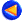### Workshop Nosevol #3

Mikael Persson : Uniqueness of minima of anharmonic oscillators.

In spectral theory of magnetic Schrödinger operators, some families of Sturm--Liouville operators often appear. For $k\geq 1$ we study the parameter-dependent family of self-adjoint operators $H(\alpha)u(t) = -u''(t)+(t^(k+1)/(k+1)-\alpha)^2u(t)$ in $L^2(\mathbb{R})$. Our aim is to show that the mapping $\mathbb{R}\ni\alpha\mapsto \lambda_1(\alpha)$ (where $\lambda_1(\alpha)$ denotes the smallest eigenvalue of $H(\alpha)$ possess a unique non-degenerate minimum. We will also discuss the connection between these operators and magnetic Schrödinger operators. This is joint work with B. Helffer and S. Fournais.